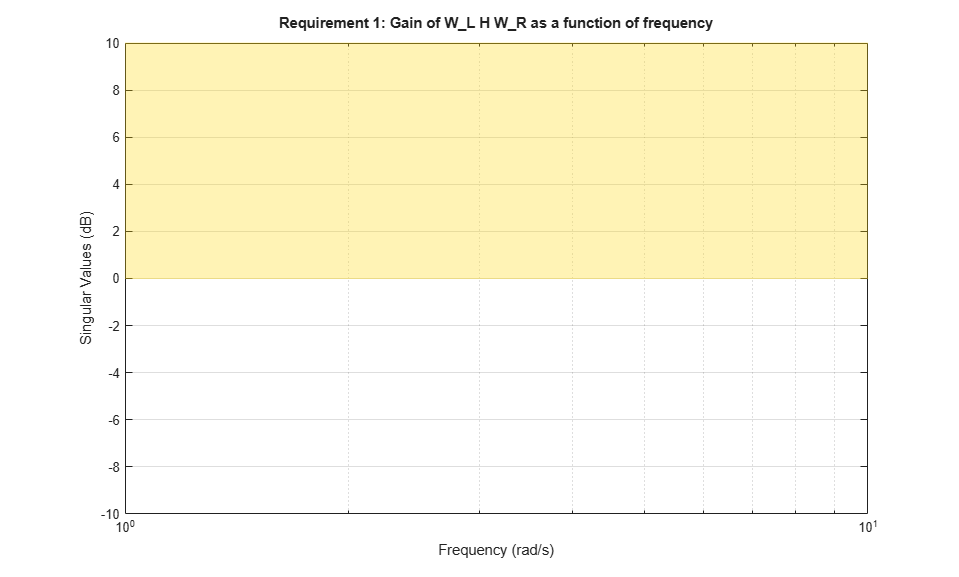Frequency-Domain Specifications

This example gives a tour of available frequency-domain requirements for control system tuning with systune or looptune.

Background

The systune and looptune commands tune the parameters of fixed-structure control systems subject to a variety of time- and frequency-domain requirements. The TuningGoal package is the repository for such design requirements.

Gain Limit

The TuningGoal.Gain requirement enforces gain limits on SISO or MIMO closed-loop transfer functions. This requirement is useful to enforce adequate disturbance rejection and roll off, limit sensitivity and control effort, and prevent saturation. For MIMO transfer functions, "gain" refers to the largest singular value of the frequency response matrix. The gain limit can be frequency dependent. For example

s = tf('s');
R1 = TuningGoal.Gain('d','y',s/(s+1)^2);

specifies that the gain from d to y should not exceed the magnitude of the transfer function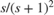.

viewGoal(R1)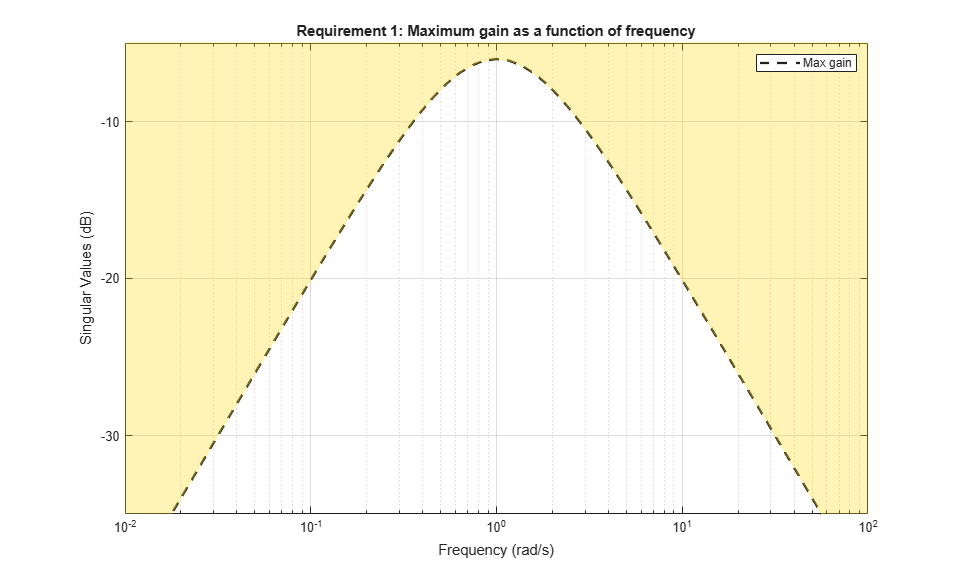It is often convenient to just sketch the asymptotes of the desired gain profile. For example, instead of the transfer function, we could just specify gain values of 0.01,1,0.01 at the frequencies 0.01,1,100, the point (1,1) being the breakpoint of the two asymptotesand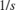.

Asymptotes = frd([0.01,1,0.01],[0.01,1,100]);
R2 = TuningGoal.Gain('d','y',Asymptotes);

The requirement object automatically turns this discrete gain profile into a gain limit defined at all frequencies.

bodemag(Asymptotes,R2.MaxGain)
legend('Specified','Interpolated')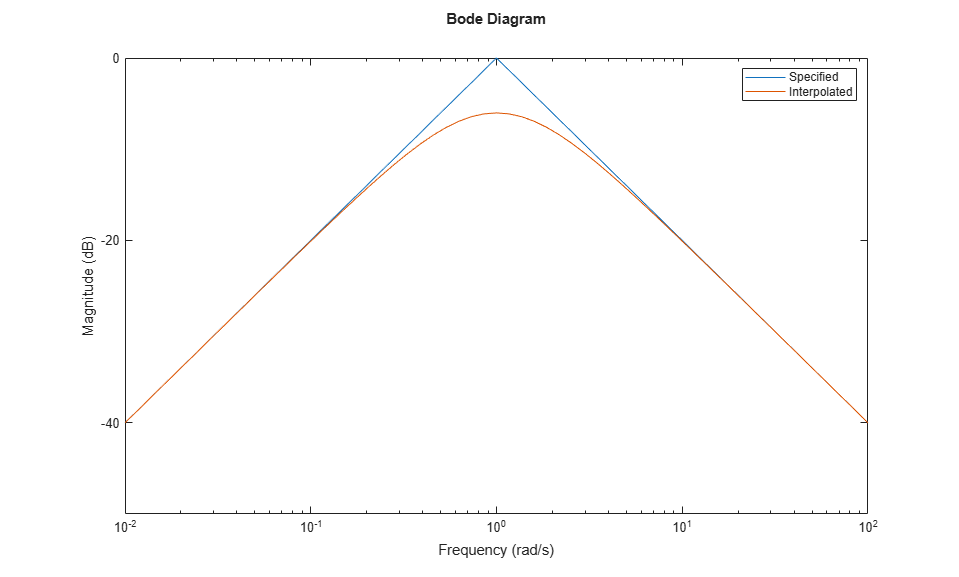Variance Amplification

The TuningGoal.Variance requirement limits the noise variance amplification from specified inputs to specified outputs. In technical terms, this requirement constrains the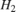norm of a closed-loop transfer function. This requirement is preferable to TuningGoal.Gain when the input signals are random processes and the average gain matters more than the peak gain. For example,

R = TuningGoal.Variance('n','y',0.1);

limits the output variance of y to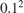for a unit-variance white-noise input n.

Reference Tracking and Overshoot Reduction

The TuningGoal.Tracking requirement enforces reference tracking and loop decoupling objectives in the frequency domain. For example

R1 = TuningGoal.Tracking('r','y',2);

specifies that the output y should track the reference r with a two-second response time. Similarly

R2 = TuningGoal.Tracking({'Vsp','wsp'},{'V','w'},2);

specifies that V should track Vsp and w should track wsp with minimum cross-coupling between the two responses. Tracking requirements are converted into frequency-domain constraints on the tracking error as a function of frequency. For the first requirement R1, for example, the gain from r to the tracking error e = r-y should be small at low frequency and approach 1 (100%) at frequencies greater than 1 rad/s (bandwidth for a two-second response time). You can use viewGoal to visualize this frequency-domain constraint. Note that the yellow region indicates where the requirement is violated.

viewGoal(R1)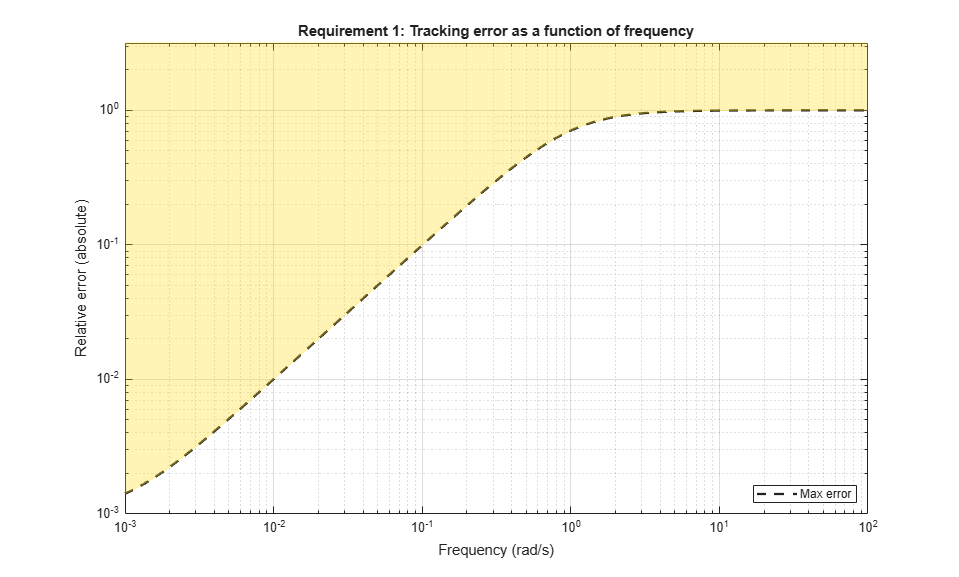If the response has excessive overshoot, use the TuningGoal.Overshoot requirement in conjunction with the TuningGoal.Tracking requirement. For example, you can limit the overshoot from r to y to 10% using

R3 = TuningGoal.Overshoot('r','y',10);

Disturbance Rejection

In feedback loops such as the one shown in Figure 1, the open- and closed-loop responses from disturbance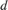to outputare related by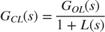whereis the loop transfer function measured at the disturbance entry point. The gain ofis the disturbance attenuation factor, the ratio between the open- and closed-loop sensitivities to the disturbance. Its reciprocal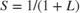is the sensitivity at the disturbance input.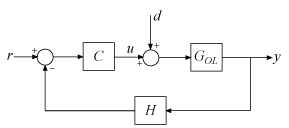Figure 1: Sample feedback loop.

The TuningGoal.Rejection requirement specifies the disturbance attenuation as a function of frequency. The attenuation factor is greater than one inside the control bandwidth since feedback control reduces the impact of disturbances. As a rule of thumb, a 10-times-larger attenuation requires a 10-times-larger loop gain. For example

R1 = TuningGoal.Rejection('u',10);
R1.Focus = [0 1];

specifies that a disturbance entering at the plant input "u" should be attenuated by a factor 10 in the frequency band from 0 to 1 rad/s.

viewGoal(R1)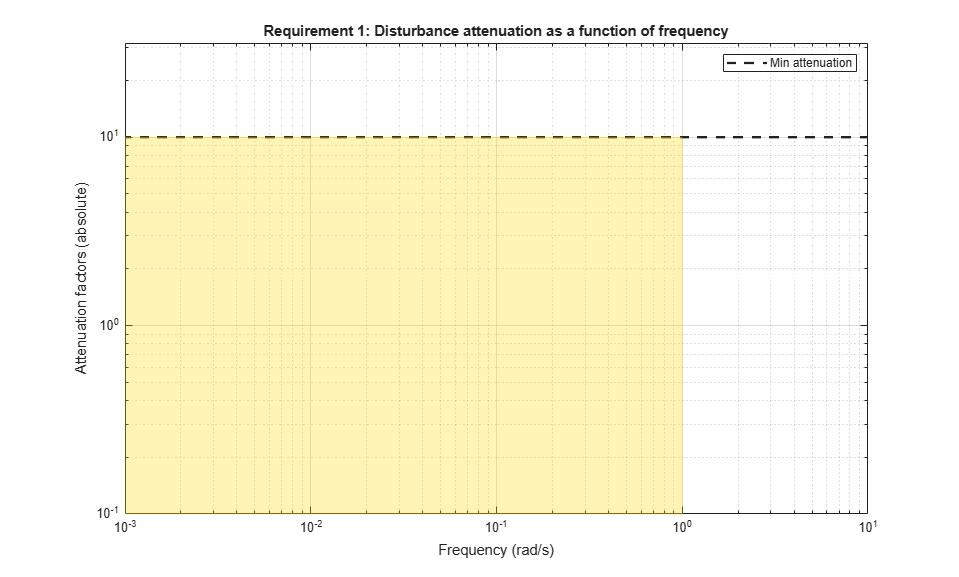More generally, you can specify a frequency-dependent attenuation profile, for example

s = tf('s');
R2 = TuningGoal.Rejection('u',(s+10)/(s+0.1));

specifies an attenuation factor of 100 below 0.1 rad/s gradually decreasing to 1 (no attenuation) after 10 rad/s.

viewGoal(R2)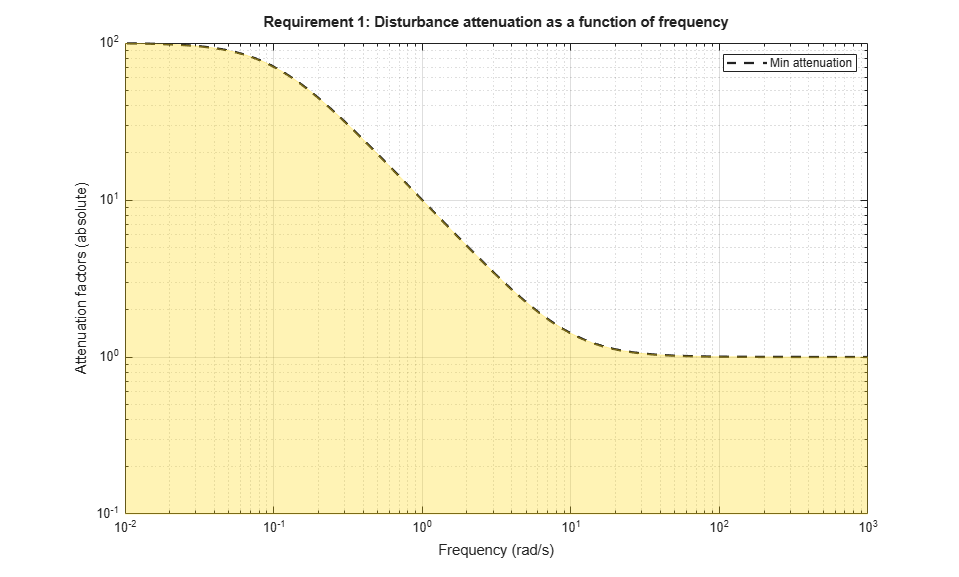Instead of specifying the minimum attenuation, you can use the TuningGoal.Sensitivity requirement to specify the maximum sensitivity, that is, the maximum gain of. For example,

R3 = TuningGoal.Sensitivity('u',(s+0.1)/(s+10));

is equivalent to the rejection requirement R2 above. The sensitivity increases from 0.01 (1%) below 0.1 rad/s to 1 (100%) above 10 rad/s.

viewGoal(R3)Frequency-Weighted Gain and Variance

The TuningGoal.WeightedGain and TuningGoal.WeightedVariance requirements are generalizations of the TuningGoal.Gain and TuningGoal.Variance requirements. These requirements constrain the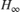ornorm of a frequency-weighted closed-loop transfer function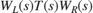, where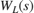and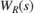are user-defined weighting functions. For example

WL = blkdiag(1/(s+0.001),s/(0.001*s+1));
WR = [];
R = TuningGoal.WeightedGain('r',{'e','y'},WL,[]);

specifies the constraint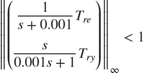Note that this is a normalized gain constraint (unit bound across frequency).

viewGoal(R)